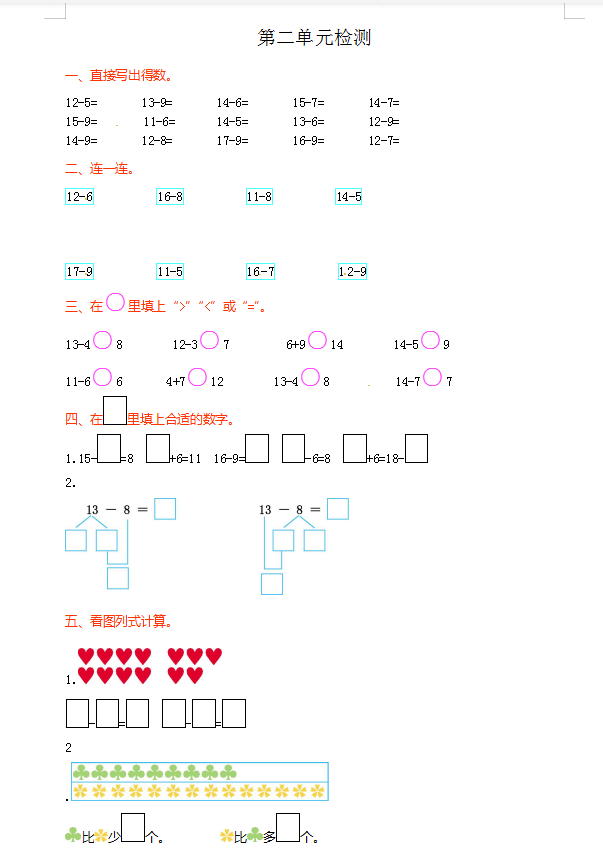12-5=　　　 13-9=　　　 14-6=　　 15-7=　　 14-7=

15-9=             11-6=　　      14-5=　　 13-6=         12-9=

14-9=　　      12-8=          17-9=　　    16-9=　     12-7=